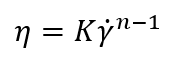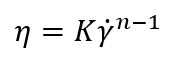# non-Newtonian Fluid Modeling: Power Law Model

November 17, 2020

Knowing how to characterize and accurately analyze non-Newtonian fluids is a vital skill when it comes to the development and distribution of these fluids. Non-Newtonian fluids are manufactured and sold in multiple industries including the chemical, oil and gas, biopharmaceutical, food and beverage, inks, paints and coatings and cosmetics industries. When characterizing non-Newtonian fluids we produce and sell, the goal is to extract important information about the fluids quickly and simply.

Scientists have been studying, analyzing, and characterizing non-Newtonian fluid behavior for decades and have developed numerous analytical models to facilitate their analysis. These mathematical model outputs can be used to interpolate data, rank, and categorize fluids, and even help make calculations for fluid dynamics simulations. One simple model used to characterize non-Newtonian fluids is the power law model (Macosko, 1994):η is the viscosity (mPa-s)
n is the power law constant (unitless)
K is the flow consistency index, (mPa-s)

The power law model, also known as the Ostwald de Waele relationship, is used to fit non-Newtonian data across shear rates where there is no evidence of a Newtonian plateau region. Fitting to the power law model is appropriate where the measured data is entirely within the shear-thinning regime across all the shear rates tested. The power law model only has two fitting constants making it much simpler than other models that fit non-Newtonian fluids such as the Cross model or Carreau-Yasuda model which contain four and five fitting constants, respectively.

Mathematically modeling your data improves your data analysis and fluid characterization. The constants generated by these fits have multiple uses from ranking fluid performance to performing calculations that are relevant for your samples. Parameters useful for fluid characterization like flow consistency index and flow behavior can be determined using this model.

Download our application note for a detailed walk through of how to perform power law fitting on non-Newtonian fluids using Microsoft Excel.Written By: Dr. Zachary Imam, RheoSense Research Scientist

## You May Also Like

These Stories on Viscosity Measurements